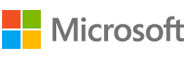New update is available. Click here to update.
Topics

# Longest Univalue Path

Moderate0/80
Average time to solve is 10m## Problem statement

You are given a binary tree, the task is to find out the length of the longest path which contains nodes with the exact same value. It is not necessary for the path to pass through the root of the binary tree.

Between two nodes, the length of the path can be defined as the number of edges contained between them.

For example, consider the following binary tree:

``````            7
/ \
7   7
/ \   \
8  3    7
``````

For the above tree, the length of the longest path where each node in the path has the same value is 3 and path is 7 -> 7 -> 7 -> 7.

Detailed explanation ( Input/output format, Notes, Images )
Constraints:
``````1 <= T <= 100
1 <= N <= 3000
-10^9 <= data <= 10^9 and data != -1

Where ‘T’ is the number of test cases, ‘N’ is the total number of nodes in the binary tree, and “data” is the value of the binary tree node.

Time Limit: 1sec
``````
Sample Input 1:
``````2
7 7 7 1 1 -1 7 -1 -1 -1 -1 -1 -1
10 3 4 3 3 -1 5 -1 -1 -1 -1 -1 -1
``````
Sample Output 1:
``````3
2
``````
Explanation of Sample Input 1:
``````            7
/ \
7   7
/ \   \
1  1    7

For the first test case, the length of the longest path where each node in the path has the same value is 3 and path is 7 -> 7 -> 7 -> 7.

10
/ \
3   4
/ \   \
3  3    5

For the second test case, the length of the longest path where each node in the path has the same value is 2 and path is 3 -> 3 -> 3.
``````
Sample Input 2:
``````2
1 4 5 4 4 -1 5 -1 -1 -1 -1 -1 -1
5 4 5 1 1 -1 5 -1 -1 -1 -1 -1 -1
``````
Sample Output 2:
``````2
2
``````Console